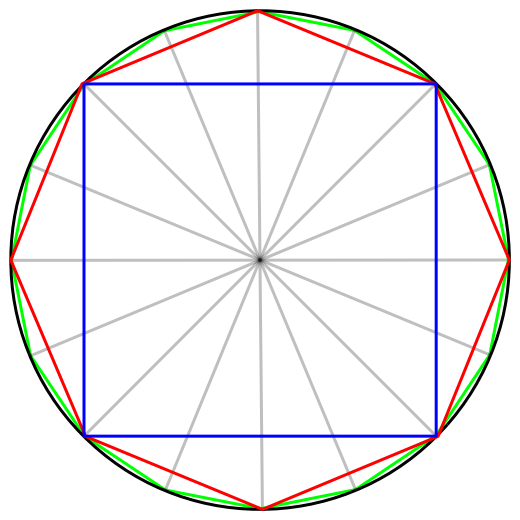Common VerticesTwo regular polygons are inscribed in the same circle. The first polygon has 1982 sides and second has 2973 sides. If the polygons have common vertices , the number of such vertices is $\text{\_\_\_\_\_\_\_\_\_\_}.$

×